The variation of acceleration of a particle executing SHM with displacement x is

1.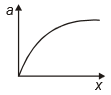2.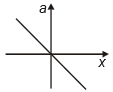3.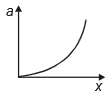4.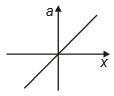Concept Questions :-

Simple harmonic motion
High Yielding Test Series + Question Bank - NEET 2020

Difficulty Level:

A particle is subjected to two simple harmonic motions in the same direction having equal amplitudes and equal frequency. If the resulting amplitude is equal to the amplitude of individual motions, the phase difference between them is:

1.  $\frac{\mathrm{\pi }}{3}$

2.  $\frac{2\mathrm{\pi }}{3}$

3.  $\frac{\mathrm{\pi }}{6}$

4.  $\frac{\mathrm{\pi }}{2}$

Concept Questions :-

Simple harmonic motion
High Yielding Test Series + Question Bank - NEET 2020

Difficulty Level:

The motion of a particle varies with time according to the relation . Then

1.  The motion is oscillatory but not SHM

2.  The motion is SHM with amplitude $a\sqrt{2}$

3.  The motion is SHM with amplitude $\sqrt{2}$

4.  The motion is SHM with amplitude a

Concept Questions :-

Simple harmonic motion
High Yielding Test Series + Question Bank - NEET 2020

Difficulty Level:

If a particle is executing SHM, with an amplitude A, the distance moved and the displacement of the body in a time equal to its period are

1.  2A, A

2.  4A, 0

3.  A, A

4.  0, 2A

Concept Questions :-

Simple harmonic motion
High Yielding Test Series + Question Bank - NEET 2020

Difficulty Level:

1.

2.

3.

4.

High Yielding Test Series + Question Bank - NEET 2020

Difficulty Level:

The displacement of a particle executing SHM is given by y = 0.25 (sin 200t) cm. The maximum speed of the particles is:

1.  200 cm/sec

2.  100 cm/sec

3.  50 cm/sec

4.  0.25 cm/sec

High Yielding Test Series + Question Bank - NEET 2020

Difficulty Level:

which of the following figure represents damped harmonic motion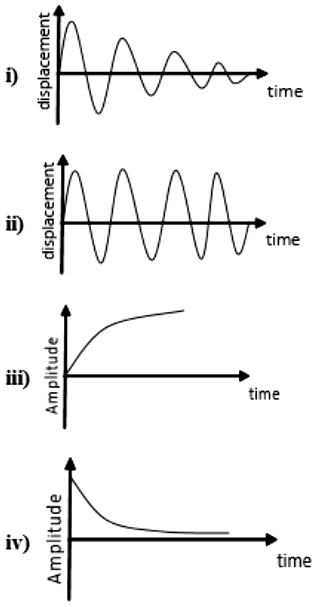1.  i and ii

2.  iii and iv

3.  i, ii, iii, and iv

4.  i, and  iv

High Yielding Test Series + Question Bank - NEET 2020

Difficulty Level:

1.

2.

3.

4.  $\frac{2\mathrm{T}}{3}$

High Yielding Test Series + Question Bank - NEET 2020

Difficulty Level:

1.

2.

3.

4.

High Yielding Test Series + Question Bank - NEET 2020

Difficulty Level:

A particle undergoes SHM with a time period of 2 seconds. In how much time will it travel from its mean position to a displacement equal to half of its amplitude?

(1) $\frac{1}{2}s$

(2) $\frac{1}{6}s$

(3) $\frac{1}{4}s$

(4) $\frac{1}{3}s$

Concept Questions :-

Simple harmonic motion